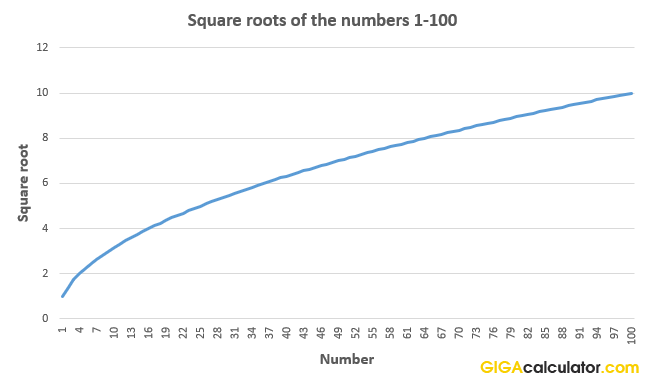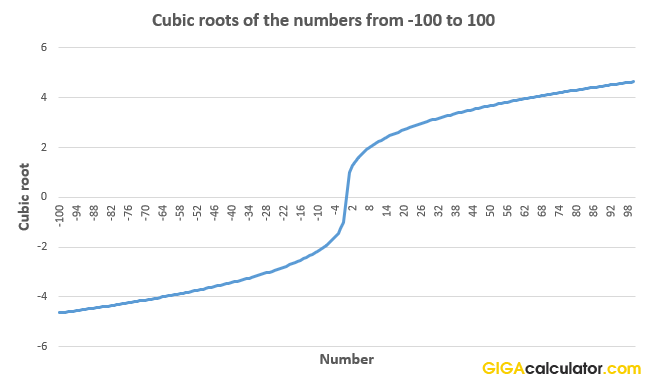# Root Calculator

Use this calculator to easily calculate the nth root of a given number.

Share calculator:

Embed this tool:
get code

## What is a root of a number?

The nth-root of a number answers the question "what number can I multiply by itself n times to get this number?". It is the reverse of the exponentiation operation where the exponent is n, so if rn = x, then we say that "r is the nth root of x". The mathematical operation of finding the root of a number has a special notation: the radical symbol √.

If n is even, then there are always two roots: a positive and a negative one, with equal value and opposite signs. The positive solution is called the principal root. If n is odd, then there is just one real number root and it has the same sign as x. That is its principal root. Some roots, e.g. the cube root, also have solutions in complex numbers and conjugates, but it is always the principal root which our root calculator will output.

The most popular root functions are the square root (n = 2) and cube root (n = 3), with the first one having an array of applications in mathematics, geometry, physics, probability theory and statistics. Cube roots have applications in angular calculations.

## Square and cube root functions

Here are visualizations of the square root and cube root functions for a small set of integers:The graphs were generated using this nth root calculator. It supports any root you may be interested in computing, to the extent that modern software allow.

## Does the calculator support fractions?

Yes, simply enter the fraction as a decimal number (use dot as a decimal separator) and you will get the corresponding root. For example, to compute the square root of 1/2 simply enter 0.5 in the number field and 2 in the root field and you will get 0.7071 as ouput. If you are having trouble converting a fraction to a decimal number, you will find our fraction to decimal converter handy.

#### Cite this calculator & page

If you'd like to cite this online calculator resource and information as provided on the page, you can use the following citation:
Georgiev G.Z., "Root Calculator", [online] Available at: https://www.gigacalculator.com/calculators/any-root-calculator.php URL [Accessed Date: 01 Apr, 2023].